# Arduino Irrigation System

In this project we want to make an automatic irrigation system with Arduino.

BeginnerFull instructions provided7,721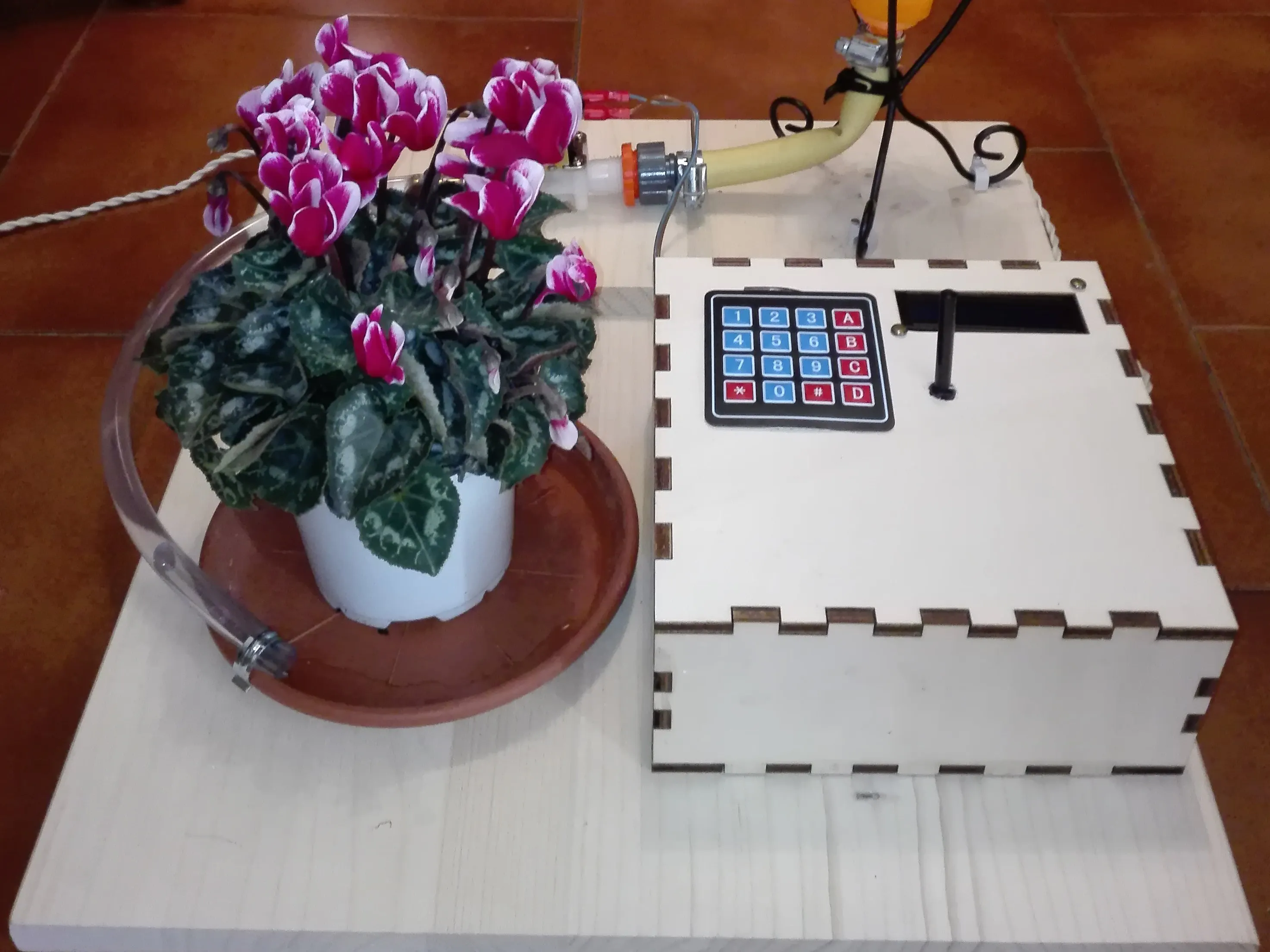## Things used in this project

### Hardware componentsArduino UNO & Genuino UNO
×1×1
×1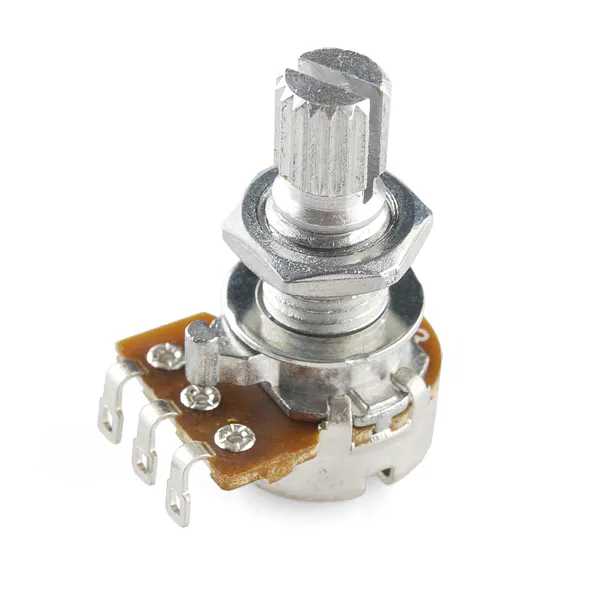Rotary potentiometer (generic)
×1
×1
 Relay (generic)
×1
×1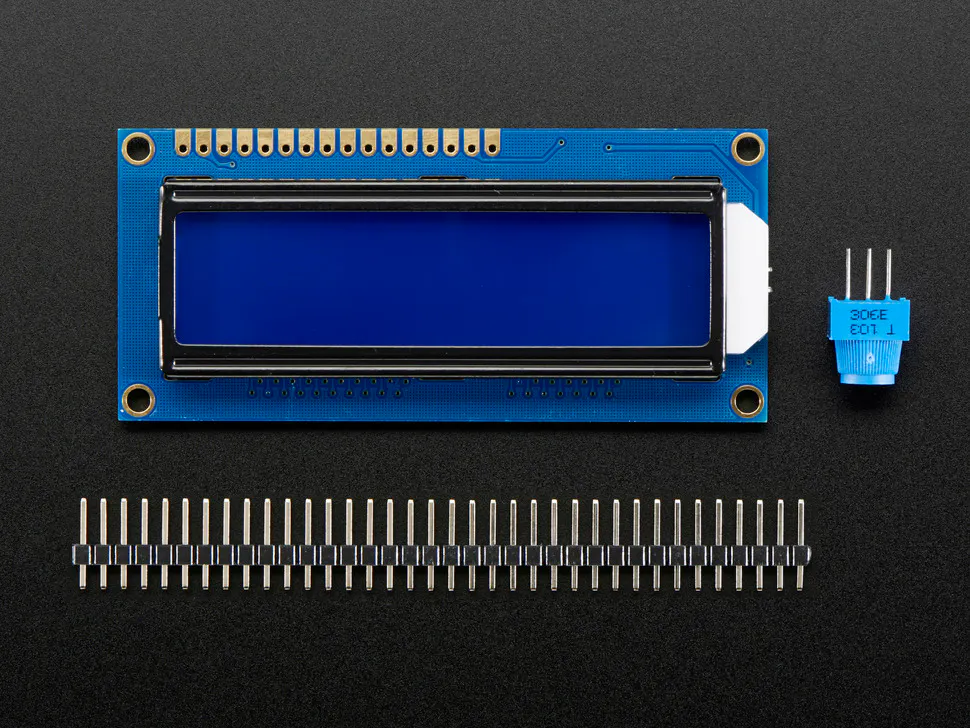Adafruit Standard LCD - 16x2 White on Blue
×1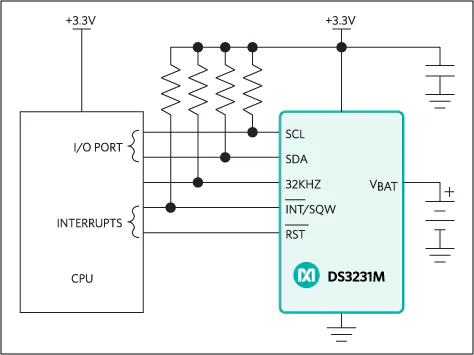Maxim Integrated DS3231M - ±5ppm, I2C Real-Time Clock
×1Resistor 10k ohm
×1

### Software apps and online servicesArduino IDE

### Hand tools and fabrication machines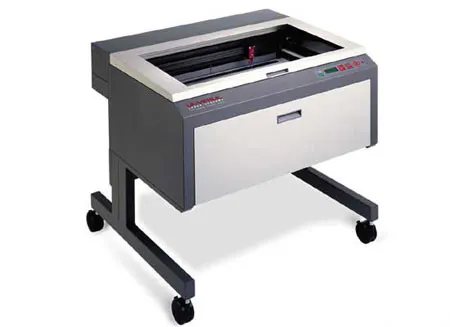Laser cutter (generic)

## Code

### Code

Arduino
```#include <Wire.h>  //real time clock sensor library
#include <DS3231.h>
#include <LiquidCrystal.h> //LCD monitor library

DS3231 clock;
RTCDateTime dt;
LiquidCrystal lcd(7, 8, 9, 10, 11, 12);   // initialize the library with the numbers of the interface pins

const byte ROWS = 4; //four rows
const byte COLS = 1; //one column
//define the cymbols on the buttons of the keypads
char hexaKeys[ROWS][COLS] = {
{'1'},
{'2'},
{'3'},
{'4'}
};
byte rowPins[ROWS] = {6, 5, 4, 3}; //connect to the row pinouts of the keypad
byte colPins[COLS] = {2}; //connect to the column pinouts of the keypad

//initialize an instance of class NewKeypad

int ThermistorPin = A0; // Temperature sensor
int relaypin = 13; //relay pin

int Vore = 0;   //time, in hours
int Vmin = 0;   //time, in minutes
int Vtemp = 0;  //limit temperature
int VOfin = 0;  //end time, in hour
int VMfin = 0;  //end time, in minutes
int Vo = 0;     //conversion

float R1 = 10000;  //temperature sensor variables
float logR2, R2, T, Tc, Tf;
float c1 = 1.009249522e-03, c2 = 2.378405444e-04, c3 = 2.019202697e-07;

void setup() {
clock.begin();
clock.setDateTime(__DATE__, __TIME__);  //set up the real time clock sensor
pinMode (ThermistorPin, INPUT);
pinMode (relaypin, OUTPUT);
lcd.begin(16, 2);   // set up the LCD's number of columns and rows:
lcd.setCursor(0, 0);     //reset the lcd monitor
lcd.print ("         ");
lcd.setCursor(0, 1);
lcd.print ("         ");
}

void loop() {
R2 = R1 * (1023.0 / (float)Vo - 1.0);
logR2 = log(R2);
T = (1.0 / (c1 + c2 * logR2 + c3 * logR2 * logR2 * logR2));
Tc = T - 273.15;
dt = clock.getDateTime();   //reading the time from the  RTC DS3231
if (customKey1 == '1' ) { // change of time
Vore = Vore + 1;
if (Vore > 23) {
Vore = 0;
}
}
if (customKey1 == '2' ) {
Vmin  = Vmin + 1;
if (Vmin > 59) {
Vmin = 0;
}
}
if (customKey1 == '3' ) { //change of temperature
Vtemp = Vtemp + 1;
if (Vtemp > 40) {
Vtemp = 0;
}
}
lcd.setCursor(0, 0);   // set the cursor to column 0, line 0
lcd.print ("Sh");      //print the time that it will start (start hour)
lcd.print (Vore);
lcd.print(":");
lcd.print(Vmin);
lcd.print("  Ch");   //print the current time (current hour)
lcd.print (dt.hour);
lcd.print (":");
lcd.print(dt.minute);
lcd.setCursor(0, 1);   // set the cursor to column 0, line 1
lcd.print ("Tl ");     //print the limit temperature
lcd.print (Vtemp);
lcd.print("C");
lcd.print(" Tm ");     //print the current temperature
lcd.print (Tc);
lcd.print("C");
if ((dt.hour == Vore) && (dt.minute == Vmin)) {   //condition for irrigation
if (Tc < Vtemp) {
digitalWrite (relaypin, HIGH);      //start irrigate
if (dt.second < 50) {
VMfin = Vmin + 2;
VOfin = Vore;        //set the final time of irrigation
if (VMfin > 60) {
VMfin = VMfin - 60;
VOfin = Vore + 1;
if (VOfin == 24) {
VOfin = 0;
}
}
delay(50000);      //delay to allow the correct calculation of the final time of irrigation, because we need to calculate it one time and not more//
}
delay(1000);
}
}
else if ((dt.hour == VOfin) && (dt.minute == VMfin)) {
digitalWrite (relaypin, LOW);   //stop irrigate at final time of irrigation
}
else {
delay(120);   //delay to refresh the LCD monitor
}
}
```

## Credits

### smana_00

0 projects • 5 followers